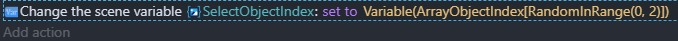# How to add probability to objects on a group

Hello, before anything thanks for your attention.
I want to create a group of objects (a lot of them) and when a button is clicked 5 Random objects are picked from the group, but i don´t want for all of them to have the same chances, i want to costumize the chance that each object have to appear. Its there any way to add a probability to each object, or something similar.

At first i made 5 variables wich have the Random numbers coming from the button and based in wich number they were, an object was created, but i had to give a range of numbers to each object and this gave me a lot problems for other features for the game, so i thought to have all of them in a group with fixed chances was better.

Thanks.

Hi!
I use this method, of course, it is not a perfect theory of probability, but it works. =)
For the sake of brevity, let’s say we have 3 objects. Further:

1. Create an array of object indices [1, 2, 3]

2. Get a random index from the array using the RandomInRange(0, 2) function (from the first element, then the length of the array minus 1). Next, we use it in the condition to get the required object.To create a certain “probability” we upgrade our array to create “probabilities” to [1, 1, 1, 1, 1, 2, 2, 3, 3, 3].

Thus, you can adjust the “frequency” of the object index in the array and, accordingly, its probability of selection. Arrays can still be mixed or somehow sorted.
To work with an array, you can use both ordinary variables (as shown in my example) and the Array Tools extension.

1 Like

Another thought came to me: there is no need to use the function of obtaining a random value. It is enough to fill the array, shuffle it and use a variable that will take into account the currently selected element (and increase it when a new value is received).
Let’s consider an example with an array `[1, 1, 1, 1, 1, 2, 2, 3, 3, 3]`:

• 50% is occupied by the object with the index “1”;
• 20% - with the index “2”;
• 30% - with the index “3”.

Shuffle the array and get, for example, `[2, 1, 1, 3, 1, 2, 3, 3, 1, 1]`. Let’s create the variable `CurrentObjectIndex = 0`. Now, when we get the element, we increase the `CurrentObjectIndex` by 1. When the value of the variable `CurrentObjectIndex` becomes greater than 9, we can shuffle the array and start over (`CurrentObjectIndex = 0`). Thus, we will get the correct frequency of occurrence of objects.

1 Like

Thanks this helped me a lot.1 Like